Time: 3 Hours                                                                                                    Max. Marks: 100

NOTE: There are 9 Questions in all.

·      Question 1 is compulsory and carries 20 marks. Answer to Q. 1 A must be written in the space provided for it in the answer book supplied and nowhere else.

·      Out of the remaining EIGHT Questions answer any FIVE Questions. Each question carries 16 marks.

·      Any required data not explicitly given, may be suitably assumed and stated.

Q.1 A    Choose the correct or best alternative in the following:                                         (2x10)

a.       The condition of equilibrium of co-planar and non-concurrent forces are

(A)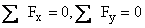(B)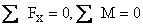(C)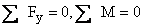(D)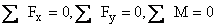b.      The example of a couple in everyday life is

(A)    Opening or closing a water tap.

(B)    turning a screw driver for tightening a screw.

(C)    opening or closing cap of water bottle.

(D)    All the above.

c.       If F is the limiting force of friction, R is the normal reaction,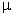is the coefficient of friction and P is the force applied, then

(A)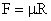(B)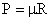(C)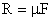(D)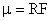d.  The polar moment of inertia of square of side a is

(A)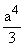(B)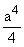(C)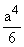(D)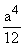e.   Two identical balls 1 and 2 are approaching each other with a velocity of U and –U respectively.  If the coefficient of restitution of both balls is 1, what will be the velocity of ball 1 after impact

(A)   2U                                               (B) U

(C) –U             (D) –2U

f.    The time of flight for a projectile projected at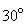to horizontal with a velocity ‘v’ is

(A)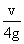(B)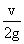(C)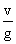(D)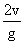g.   A cage of lift of weight 10 kN starts from rest and moves downwards with a constant acceleration of 0.1g.  What is the tension in the cable to which the cage is tied during movement?

(A)  4 kN                                            (B)  4.5 kN

(C)  5 kN                                            (D)  5.5 kN

h.   A simply supported horizontal beam is subjected to vertical downward loads on the top surface.  What is the nature of stress on the bottom surface of the beam?

(A)  Tensile stress                                (B)  Compressive stress

(C)  Shear stress                                  (D)  a combination of compressive stress

and shear stress

i.  The maximum bending moment on simply supported beam of length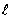subjected to a concentrated load W at the centre is

(A)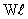(B)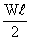(C)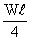(D)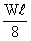j.    Every cross-section of a shaft subjected to pure torsion is under

(A)  shear stress                                  (B)  compression stress

(C)  tensile stress                                 (D)  bending stress

Answer any FIVE Questions out of EIGHT Questions.

Each question carries 16 marks.

Q.2     a.   State the law of polygon of forces.                                                                      (2)

b.   A rigid prismatic bar AB 4m long is supported in a vertical plane by a hinge at the end A and by a horizontal string attached to its middle point C.  The end B of bar carries a load of 200 N as shown in Fig.1.  Find the tension in the string and reaction and its direction.                                              (14)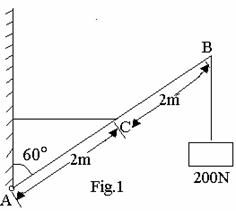Q.3     a.   Define limiting force of friction and coefficient of friction.                                     (2)

b.   A block lying over a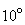wedge which is on a horizontal floor and touching a vertical wall weighs 3.0 kN.  It is to be raised by applying a horizontal force to the wedge as shown in Fig.2. Assuming the coefficient of friction between all surfaces in contact to be 0.3, find, the minimum horizontal force to be applied for raising the block.                                            (14)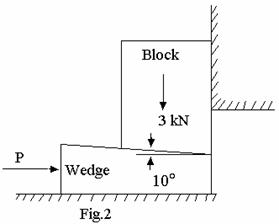Q.4     a.   State the three Newton’s laws of motion.                                                            (3)

b.   A flywheel is rotating at 500 rpm and after 10 seconds it is rotating at 400 rpm.  If the retardation is uniform, determine the number of revolutions made by the flywheel and time taken before it comes to rest from the speed of 500 rpm.                                                                                           (13)

Q.5     a.   The time of sliding down a smooth inclined plane is twice that of falling down the vertical height of the plane.  Find the inclination of the plane to the horizontal.                                                               (8)

b.   Two masses of equal mass m are connected by a string passing over a smooth pulley.  What mass must be removed from one and added to the other, so that the system may describe 88.29 m is 6 seconds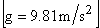.                                                                                               (8)

Q.6     a.    Define relative motion.                                                                                       (2)

b.   A policeman travelling in a train which is moving at a speed of 72 km/hr, fires at a person moving away from the train at right angles to the train with a speed of 54 km/hr.  The line connecting the policeman and the person makes an angle of 400 to the train at the time of shooting.  If the bullet velocity is 680 km/hr, find the angle at which he should aim in order to hit the person.                                                       (14)

Q.7    a.   Define elastic limit and yield point.                                                                      (2)

b.   A 2m long steel bar is having a uniform diameter of 40 mm for a length of 1 m and the remaining 1m length gradually tapers from the diameter of 40 mm to a diameter of 20 mm.  Determine the elegation of the bar when subjected to an axial tensile load of 160 kN.  Take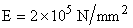. (14)

Q.8           Derive the bending equation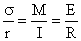stating all the assumptions made for proving it and define section modulus.                                              (15+1)

Q.9     a.   Derive an expression for the power transmitted by shaft running at ‘N’ rpm in terms of mean torque ‘T’ N-m.                                                                                                                      (3)

b.   A solid circular shaft is to transmit 450 kW at 400 rpm.  If the maximum shear stress is not to exceed 80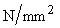, find the shaft diameter.  Find the width and depth of the key and number of both required to connect this shaft with a coupling to another shaft.  The bolt circle diameter is 300 mm.  The allowable shear stress in the key and bolt is also 80and allowable compression stress in the key is 200.  The length of key is 150 mm.                                                                            (13)